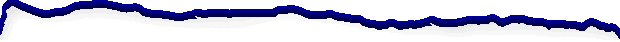The Pythagorean Theorem and Related Topics Here I will post some fun things connected to the Pythagorean Theorem. Some of the things ready to post are a note about the radius of the circle inscribed in a triangle with integral sides. There is an animated illustration of Bhaskara's proof of the Pythagorean Theorem. I have been told that somewhere there is a model of the Pythagorean Theorem built out of glass or plastic so that the squares on the sides and hypotenuse can hold a colored liquid. The liquid is then allowed to flow into the large square on the hypotenuse and demonstrates that the two squares exactly fill the lower square. This is no proof, but it is a nice demonstration. Rather than build an actual model, I created an animated gif graphic model. to show how this might look. Click here to view this demonstration. Another animation I have is an animated demonstration of Euclid's proof for the Pythagorean theorem. Click here to view this demonstration. It's here! A note on generating primative Pythagorean triples.